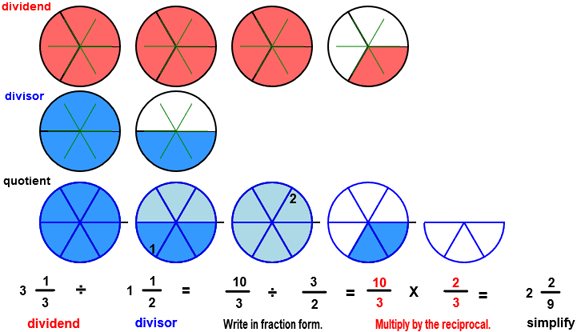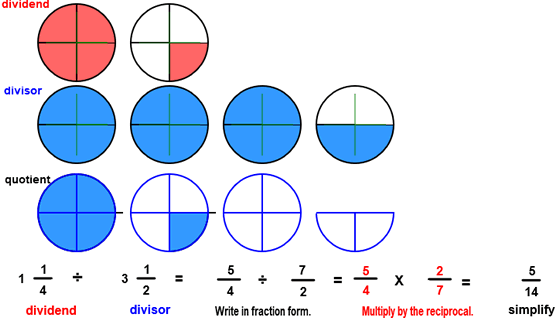# Divide and simplify fractions

## Divide Fractions and Simplify with Circle Models

DIVIDE AND SIMPLIFY
CORRECT:
ATTEMPTS:
SCORE:
PERCENT
WHOLE
NUMERATOR
DENOMINATOR

# DIVIDE AND SIMPLIFY FRACTIONS INSTRUCTIONS

Follow the directions in the dialog box after pressing the <START> button. The <EXPLAIN> button may then be pressed after you enter the divisor to see how to do the example.

The following image was made by this program.Divide Fractions and Simplify is similar to the previous program DIVIDE FRACTIONS except that the divisor may be larger than the dividend and the quotient may be a mixed number.

You can see from the image that 2 29 of the divisor will fit into the dividend. Notice in the quotient that two (2) complete divisors and 29 of a divisor will fit into the dividend.The image changes in shades of blue when a complete divisor fits in.The above  image was made from Divide Fractions With Circles Designe r:

The divisor is larger than the dividend in the above image so only part of the divisor will fit into the dividend. The quotient shows that 514 of the divisor fits into the dividend.

To calculate the quotient first write the dividend and the divisor in fraction form as shown in the example above. Then multiply 54 by the reciprocal of the divisor. The reciprocal of the divisor is found by  replacing the numerator with the denominator and replacing the denominator with the numerator. In short, to divide by 72 multiply by the reciprocal 27.

Another important idea: When the divisor is smaller than the dividend, the quotient is larger than 1. When the divisor is equal to the dividend, the quotient is equal to 1. When divisor is larger than the dividend, the quotient is smaller than 1.

See the program MIXED NUMBERS for information on writing fractions in mixed form.

See the program RENAME IN LOWEST TERMS for information on writing fractions in lowest terms.

See MULTIPLY FRACTIONS  for instructions on how to multiply fractions.

See MULTIPLICATIVE INVERSE for more information on how to find the inverse or reciprocal.

The quotient must be written in simplest form as a mixed number and in lowest terms, if necessary.

For more instruction on dividing fractions go to How To Divide Fractions.

After you enter the quotient you may press the <REPORT> button. The report will ask for your name but you may submit a code for your name. This report will give the same results as on the dialog box. The report may be printed or e-mailed.